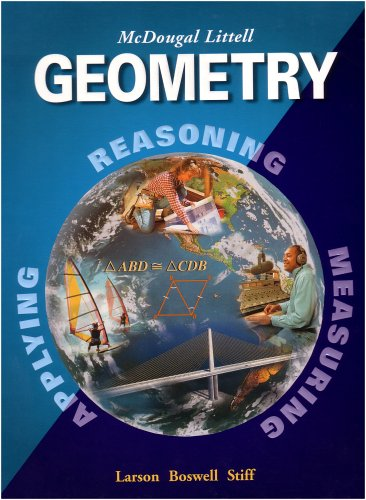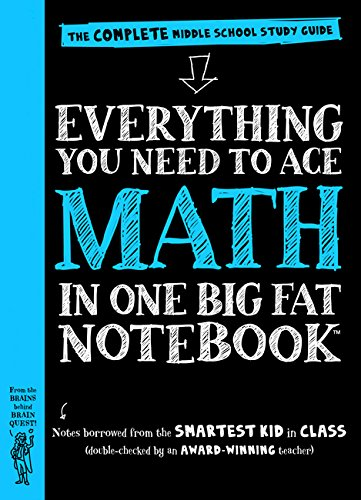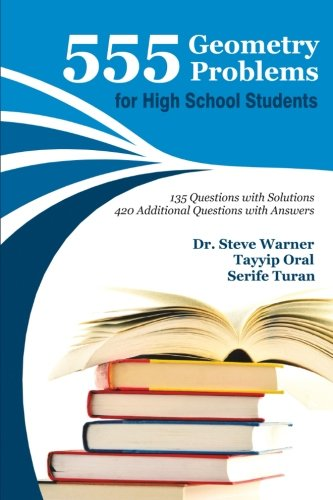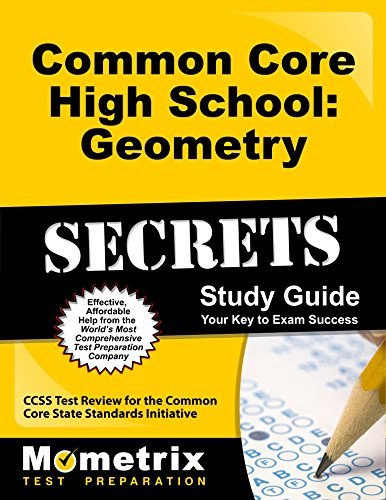# Best Geometry Books for High School | Improve Mathematical Knowledge

These books are full of good examples and thorough explanations of how to do problems. Exercises, and solutions throughout, plus hundreds of drawings, graphs, and tables will help you to pass the exam. Here you will get some of the best geometry books for high school.

## 1. Geometry, Grades 9-12: Mcdougal Littell High School Math (McDougal Littell High Geometry)Author: Ron Larson,Laurie Boswell,Lee Stiff
Published at: 01/01/2004
ISBN: 0618250220

Geometry, Grades 9-12 is a well-organized book with good examples and thorough explanations of how to do problems. The text is easy to understand for high school learners.

This book gives you

• Self-explanatory and simple answers and explanations at the end of the textbook
• Online quizzes and tests
• "Key Concepts" part
• Well written and thoroughly explained theorems and postulates
• Standardized test examples
• Problem sets and practice quizzes will reinforce the material
• Perfect CD for high schoolers or adult learners.

## 2. Tutor in a Book's Geometry

This book is recommended to those who need extra help with geometry, or individuals, who need assistance in a subject that they last encounter during their own high school days.

This book gives you

• Diagrams that show key ideas, shortcuts, common mistakes to avoid, and explanations.
• Theorems, axioms, and proofs for refreshing your memory
• The idea to do all the problems
• Visual aids and encouraging tone appeal to kids so they read it.
• More than 500 of the right, well-illustrated, carefully worked out and explained proofs and problems.
• Dozens of graphic organizers, which help students understand, remember and recognize the connections between concepts.

Tutor in a Book's Geometry provides services as a skilled private tutor. It provides the most effective solutions to all the geometric problems.

## 3. Painless Geometry (Painless Series)

This book is well recommended to middle school and high school students who are having a difficult time with a specific subject.

You'll learn

• How geometric principles can solve real-world problems
• How to experience the math
• The number of hands-on activities.

Important coverage are

• A painless beginning
• Angles
• Parallel and perpendicular lines
• Triangles
• Similar and congruent triangles
• Circles
• Perimeter, area, and volume
• Graphing.

## 4. Everything You Need to Ace Math in One Big Fat Notebook: The Complete Middle School Study Guide (Big Fat Notebooks)Author: Workman Publishing,Altair Peterson
Published at: 09/08/2016
ISBN: 0761160965
Everything You Need to Ace Math in One Big Fat Notebook is a complete middle school study guide and contains lots of important topics for high school students.

It covers many useful topics including factors and greatest common factors, multiples and least common multiple, unit rate and unit price, proportions, scientific notation, square and cube root, the Pythagorean theorem, circles, circumference, and area, linear equations and functions and much more.

This book is consists of the following 6 units

1. The number system
2. Ratios, Proportions, and Percents
3. Expressions and equations
4. Geometry
5. Statistics and probability
6. The coordinate plane and functions.

## 5. E-Z Geometry (Barron's E-Z Series)

E-Z Geometry provides math with charts, graphs, diagrams, instructive line illustrations, and appropriate, amusing cartoons. It also covers the "how" and "why" of geometry, with examples, exercises, and solutions throughout, plus hundreds of drawings, graphs, and tables.

• Building a geometry vocabulary
• Measure and congruence
• Angle pairs and perpendicular lines
• Parallel lines
• Angles of a polygon
• Proving triangles are congruent
• Applying congruent triangles
• Geometric inequalities
• Ratio, proportion, and similarity
• The right triangle
• Circles and angle measurement
• Chord, tangent, and secant segments
• Area and volume
• Coordinate geometry
• Locus and constructions
• Transformation geometry.

## 6. Concise Geometry: Master Geometry with 30 Hours of Self Study

Concise Geometry is a great companion tool for current geometry students. The lessons are quick, easy to understand which makes it must have for students. It is like a tutorial that can be used for geometry self-study, as a geometry workbook, as a geometry tutor, or as a higher-quality replacement for geometry for dummies. You can learn common core high school geometry after only 30 hours of study.

It contains

• Many important topics to give you a clear idea about, cosine, the law of sines, volume, etc.
• Difference between congruent, complementary, and supplementary angles
• Geometry topics to meet the needs of modern high school students.

## 7. HIGH SCHOOL MATH 2015 COMMON CORE GEOMETRY STUDENT EDITION GRADE 9/10

This is an excellent resource book for both parents and students. The examples and explanations are good. The author Prentice Hall provides answers for odd numbered questions in the back of the book so you can check your accuracy and understanding.

There are

• Examples with explanation
• Transformations after triangle congruence and similarity

## 8. 555 Geometry Problems for High School Students: 135 Questions with Solutions, 420 Additional Questions with AnswersAuthor: Steve Warner,Tayyip Oral,Serife Turan
Published at: 27/03/2015
ISBN: 1511432411
This guide includes many features summaries of rules and formulas, as well as plenty of sample questions. This is a fun read for those who want to refresh their math skills. The answer key and practice test will help to do better.

Coverage includes

• Introduction
• Angles
• Angles in a triangle
• Comparing sides and angles in a triangle
• The Pythagorean theorem and it's converse
• Isosceles right triangle
• The perimeter of the triangle
• 30°, 60°, 90° triangle
• Median of a triangle
• The angle bisector of a triangle
• The altitude of a triangle
• Equilateral triangle
• Rectangular prisms
• Cubes
• Triangular prisms
• Pyramids
• Cylinders
• Cones
• Spheres and much more.

555 Geometry Problems for High School Students also contains
• 130+ questions with solutions
• 28 tests which will definitely help students to understand the geometric concepts better
• The most effective methods, tips, and strategies for solving geometry problems in both conventional and unconventional ways

## 9. Common Core High School: Geometry Secrets Study Guide: CCSS Test Review for the Common Core State Standards InitiativeAuthor: CCSS Exam Secrets Test Prep Team
Published at: 14/02/2013
ISBN: 1627330402

The Common Core High School: Geometry Exam is extremely challenging, and thorough test preparation is essential for success. In this book, CCSS Exam Secrets Test Prep Team provides specific and detailed information that will be key to passing the Common Core High School: Geometry Exam. Practice questions with detailed answer explanations will help to move forward in the geometry field.

There are 5 sections which cover the following

The Congruence section covers:

• Point and line
• Dilation
• Compass and a straightedge

The Similarity, Right Triangles, and Trigonometry section covers:

• Proving the Pythagorean Theorem using similar triangles
• Using cosine to solve problems involving right triangles
• Using the Law of Sines and Cosines

The Circles section covers:

• Construction of a circle inscribed in a triangle
• Deriving the formula for the area of a sector

The Expressing Geometric Properties with Equations section covers:

• Identify the equation of a parabola given a focus and directrix
• Using coordinates to find the area of a figure

The Geometric Measurement and Dimension section covers:

• A formula for the area of a circle
• The formula for the volume of a cone
• Cavalieri's principle.

Thanks for reading this post. If you have any opinion don't hesitate to comment here. Also please subscribe our newsletter to get more updates.